SYLLABUS  Previous: 1.2.2 Partial differential equations  Up: 1.2 Differential Equations  Next: 1.2.4 Characteristics and dispersion

### 1.2.3 Boundary / initial conditions

Slide : [ BC/IC intial conditions - boundary conditions || VIDEO login]

Depending on the problem, initial conditions (IC)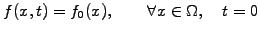(1.2.3#eq.1)

and / or boundary conditions (BC) need to be imposed. The latter are often of the form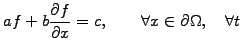(1.2.3#eq.2)

and are called Dirichlet (b=0), Neumann (a=0), or Robin (c=0) conditions. Other forms include the periodic condition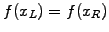with {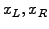}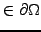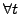, and the outgoing-wave conditions if the domain is open. To prevent reflections from the computational boundary of an open domain, it can be useful to introduce absorbing boundary conditions: the implementation depends on the numerical method that is being used, but typically model small layers of artificial material that absorbs the outgoing wave using a few mesh cells. Perfectly matched layers (PML)  are particularly useful for electromagnetic wave problems and will be discussed in conjunction with the leap-frog method (exercise 2.08).

SYLLABUS  Previous: 1.2.2 Partial differential equations  Up: 1.2 Differential Equations  Next: 1.2.4 Characteristics and dispersion